# Interoperability testing for hyperparameter tuning: MLflow, LightGBM, sklearn, and dask-ml

MLflow autologging allows monitoring LightGBM training loss during model training. This behavior is not always expected when we use scikit-learn and dask to tune LightGBM models. This notebook describes how the unexpected behavior manifests and explains some gotchas when using these tools together.
ML
MLOps
MLflow
Interoperability
Published

March 17, 2023

There are numerous open source ML packages in python ecosystem. Developers do their best to maximize interoperability in relation to other main ML packages but it’s not possible to check every possible combination. That’s why I think some of the responsibility of interoperability lies on users. MLflow’s autologging method is quite handy because with a single line of code (`mlflow.autologging`), we obtain useful metrics of model behavior such as confusion matrix, feature importance, or training loss over epochs. However, this is not always guaranteed when we apply model tuning on top by using scikit-learn and dask.

In this notebook, I first demonstrated what MLflow autologging method did particularly for LightGBM models. Then, I tried the same autologging in model tuning frameworks of scikit-learn and Dask-ML backend, and how the autologging method behaves. Check `environment.yml` to run the notebook.

``````import numpy as np
import lightgbm as lgb

import mlflow
from mlflow.client import MlflowClient

from sklearn import datasets
from sklearn.model_selection import train_test_split, RandomizedSearchCV, PredefinedSplit
from sklearn.pipeline import Pipeline
from sklearn.metrics import log_loss, roc_auc_score

from distributed import Client

seed = 97531

%watermark -d -t -u -v -g -r -b -iv -a "Hongsup Shin"``````
``````Author: Hongsup Shin

Last updated: 2023-03-19 23:00:45

Python implementation: CPython
Python version       : 3.9.16
IPython version      : 8.10.0

Git hash: 0eaf0c3c88c3e909b76c36af9b13fea1f04d7c08

Git repo: https://github.com/hongsupshin/hongsupshin.github.io.git

Git branch: 1-mlflow

lightgbm: 3.3.2
sklearn : 1.2.0
numpy   : 1.24.0
mlflow  : 2.1.1
``````## Set up

### MLflow

MLflow comes with a tracking UI, which you can launch by running `mlflow ui`. By default, you can see the UI http://localhost:5000. Here, I assumed that you ran `mlflow ui` before the following cell where experiment location was defined.

``````mlflow.set_tracking_uri("http://127.0.0.1:5000")
mlflow.set_experiment("mlflow_tune_demo")``````
``<Experiment: artifact_location='mlflow-artifacts:/618881380489654419', creation_time=1679103932467, experiment_id='618881380489654419', last_update_time=1679103932467, lifecycle_stage='active', name='mlflow_tune_demo', tags={}>``

`mlflow.autolog()` should be called before running training but this enables all supported libraries that are imported. Thus, specific autologging is recommened:

``mlflow.lightgbm.autolog()``

### Data and model

For this walkthrough, I used the breast cancer dataset from scikit-learn (`sklearn.datasets.load_breast_cancer()`), which is a binary classificaiton problem. For training, I split the dataset into train (50%), validation (25%) and test sets (25%). The validation set was used for model tuning.

``````breast_cancer = datasets.load_breast_cancer()
X = breast_cancer.data
y = breast_cancer.target

X_train_val, X_test, y_train_val, y_test = train_test_split(X, y, test_size=0.25, random_state=seed)
X_train, X_val, y_train, y_val = train_test_split(X_train_val, y_train_val, test_size=0.33, random_state=seed)

train_set = lgb.Dataset(X_train, label=y_train)
valid_set = lgb.Dataset(X_val, label=y_val)``````

Instead of `lightgbm.LGBMClassifier`, the scikit-learn API, we use the native LightGBM (`lightgbm.train`).

## LightGBM autologging for a single training run (no tuning)

To test the limits of autologging and make things more interesting, I set up the following: - Apply an early-stopping callback - Track two types of metrics: log-loss (`"binary_logloss"`) and AUROC (`"auc"`) - Track two types of datasets: training and validation - Log test metrics in addition to the autologged metrics using `mlflow.log_metrics`

and passed artbitrary hyperparameter values.

``````params = {
"objective": "binary",
"metric": ["binary_logloss", "auc"],
"learning_rate": 0.1,
"subsample": 1.0,
"seed": seed,
"num_iterations": 10,
"early_stopping_round": 5,
"first_metric_only": True,
"force_col_wise":True,
"verbosity": -1,
}``````
``````with mlflow.start_run(run_name="lgb_single") as run:

model = lgb.train(
params=params,
train_set=train_set,
callbacks=[lgb.early_stopping(stopping_rounds=5), lgb.log_evaluation()],
valid_sets=[train_set, valid_set],
valid_names=["train", "val"],
)

y_pred_proba = model.predict(X_test)
loss = log_loss(y_test, y_pred_proba)
roc_auc = roc_auc_score(y_test, y_pred_proba)

mlflow.log_metrics(
{
"test-logloss":loss,
"test-auc": roc_auc,
}
)``````
``````/opt/anaconda3/envs/mlflow_tune/lib/python3.9/site-packages/lightgbm/engine.py:177: UserWarning: Found `num_iterations` in params. Will use it instead of argument
_log_warning(f"Found `{alias}` in params. Will use it instead of argument")``````
`````` train's binary_logloss: 0.587637    train's auc: 0.986343   val's binary_logloss: 0.577365  val's auc: 0.942092
Training until validation scores don't improve for 5 rounds
 train's binary_logloss: 0.525352    train's auc: 0.989106   val's binary_logloss: 0.52163   val's auc: 0.963598
 train's binary_logloss: 0.473652    train's auc: 0.990591   val's binary_logloss: 0.47546   val's auc: 0.978383
 train's binary_logloss: 0.427402    train's auc: 0.992129   val's binary_logloss: 0.428912  val's auc: 0.983647
 train's binary_logloss: 0.388029    train's auc: 0.994553   val's binary_logloss: 0.392357  val's auc: 0.985551
 train's binary_logloss: 0.355106    train's auc: 0.995543   val's binary_logloss: 0.361549  val's auc: 0.986335
 train's binary_logloss: 0.323011    train's auc: 0.996247   val's binary_logloss: 0.330934  val's auc: 0.990031
 train's binary_logloss: 0.297144    train's auc: 0.996247   val's binary_logloss: 0.309573  val's auc: 0.990255
 train's binary_logloss: 0.272297    train's auc: 0.996508   val's binary_logloss: 0.286207  val's auc: 0.990927
    train's binary_logloss: 0.250728    train's auc: 0.996455   val's binary_logloss: 0.265777  val's auc: 0.991823
Did not meet early stopping. Best iteration is:
    train's binary_logloss: 0.250728    train's auc: 0.996455   val's binary_logloss: 0.265777  val's auc: 0.991823``````
``2023/03/19 23:00:57 WARNING mlflow.utils.autologging_utils: MLflow autologging encountered a warning: "/opt/anaconda3/envs/mlflow_tune/lib/python3.9/site-packages/_distutils_hack/__init__.py:33: UserWarning: Setuptools is replacing distutils."``

When training was done, the UI showed the autologged metrics such as feature importance scores and plots: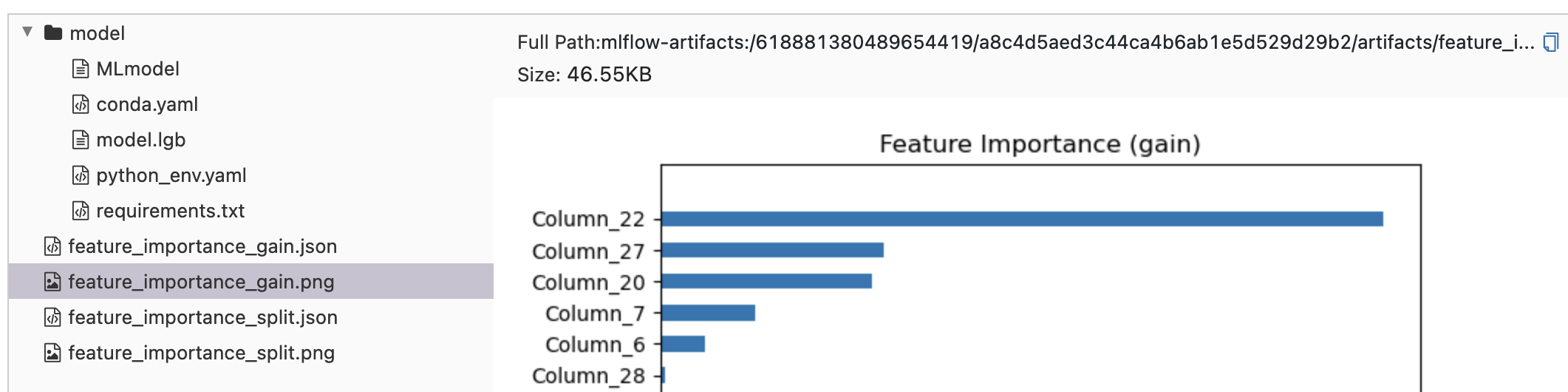The UI also shoed other metrics I defined when setting up the training. This information is under “Metrics” section in the run. When I selected `train-binary_logloss`, it showed a log-loss vs. iteration curve. I could overlay `val-binary_logloss` on top of it, which would be useful to identify model overfitting.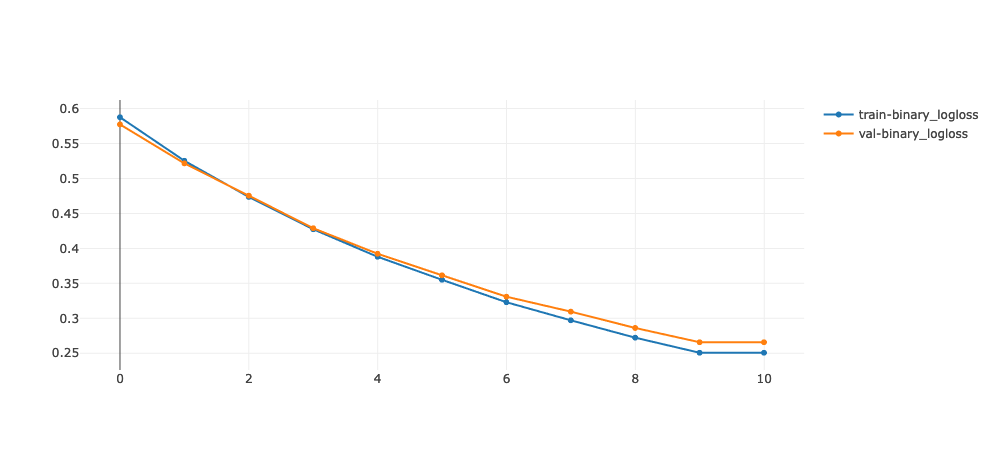I could fetch all logged metrics via `mlflow.client.MlflowClient`.

``````mlflow_client = MlflowClient()
run_id = run.info.run_id
mlflow_run = mlflow_client.get_run(run_id)
print(mlflow_run.data.metrics)``````
``{'train-auc': 0.9964553794829024, 'train-binary_logloss': 0.2507277280941103, 'val-binary_logloss': 0.2657767821072834, 'test-auc': 0.9735537190082645, 'stopped_iteration': 10.0, 'val-auc': 0.9918234767025089, 'best_iteration': 10.0, 'test-logloss': 0.30723647532041254}``

This confirms that with `mlflow.lightgbm.autolog`, the following metrics were logged in the UI: - Optimization loss over iterations - Metrics from train and validation datasets - Feature importance scores and plots - Additional metrics logged by `mlflow.log_metrics`

## Hyperparameter tuning and MLflow autologging

After some testing, I learned that the autologging behavior changed depending on tuner and autologging types. I tested scikit-learn and LightGBM autologging, and scikit-learn and Dask-ML tuners. This resulted in the following four combinations to test:

Test # LightGBM autologging scikit-learn autologging Tuner backend
1 No Yes scikit-learn
3 Yes Yes scikit-learn

### Test 1. sklearn autolog and sklearn tuner

To reduce the interaction btw `mlflow.lightgbm.autolog` and `mlflow.sklearn.autolog`, I turned the former first.

``mlflow.lightgbm.autolog(disable=True)``
``mlflow.sklearn.autolog(max_tuning_runs=None) # log all runs``

Here, I also used `PredefinedSplit` instead of k-fold to match the datasets for a hyperparameter search and evaluation parameters in LightGBM.

``````X_train_val, X_test, y_train_val, y_test = train_test_split(X, y, random_state=seed)

n_val_size = 100
n_train_size = X_train_val.shape - n_val_size
ps = PredefinedSplit(test_fold=*n_val_size + [-1]*n_train_size)

for train_index, val_index in ps.split():
X_train = X_train_val[train_index, :]
X_val = X_train_val[val_index, :]
y_train = y_train_val[train_index]
y_val = y_train_val[val_index]``````

Additionally, to be consistent with the autologging and tuner types, I used the scikit-learn API version of LightGBM (`LGBMClassifier`). For this tuning example, I chose `learning_rate` and `subsample` hyperparameters.

``````n_search = 3

with mlflow.start_run(run_name="test_1") as run:

clf = lgb.LGBMClassifier(
objective="binary",
metric="binary_logloss",
seed=seed,
class_weight="balanced",
n_estimators=10,
)

pipe = Pipeline([("clf", clf)])
param_space = {
"clf__learning_rate": np.linspace(0.05, 0.1, 10),
"clf__subsample": np.linspace(0.1, 1, 10),
}

search_cv = RandomizedSearchCV(pipe, param_space, cv=ps, n_iter=n_search)
search_cv.fit(
X_train_val,
y_train_val,
clf__eval_set=[(X_val, y_val)],
clf__eval_names=['val'],
clf__eval_metric=['binary_logloss'],
)``````
`````` val's binary_logloss: 0.624749
 val's binary_logloss: 0.568357
 val's binary_logloss: 0.520761
 val's binary_logloss: 0.480421
 val's binary_logloss: 0.442099
 val's binary_logloss: 0.410214
 val's binary_logloss: 0.382292
 val's binary_logloss: 0.357785
 val's binary_logloss: 0.335433
    val's binary_logloss: 0.31392
 val's binary_logloss: 0.637054
 val's binary_logloss: 0.589451
 val's binary_logloss: 0.547508
 val's binary_logloss: 0.511241
 val's binary_logloss: 0.478765
 val's binary_logloss: 0.448812
 val's binary_logloss: 0.423736
 val's binary_logloss: 0.398193
 val's binary_logloss: 0.377066
    val's binary_logloss: 0.356542
 val's binary_logloss: 0.653833
 val's binary_logloss: 0.618878
 val's binary_logloss: 0.586995
 val's binary_logloss: 0.558061
 val's binary_logloss: 0.531579
 val's binary_logloss: 0.507355
 val's binary_logloss: 0.485109
 val's binary_logloss: 0.463636
 val's binary_logloss: 0.444977
    val's binary_logloss: 0.427695
 val's binary_logloss: 0.620853
 val's binary_logloss: 0.56225
 val's binary_logloss: 0.512275
 val's binary_logloss: 0.464753
 val's binary_logloss: 0.426894
 val's binary_logloss: 0.390463
 val's binary_logloss: 0.358157
 val's binary_logloss: 0.330555
 val's binary_logloss: 0.305165
    val's binary_logloss: 0.282671``````

The UI showed that 1 parent run and `n_search` (3) child runs were created, where the parent run had the autologged metrics such as confusion matrix, ROC curve, and PR curve: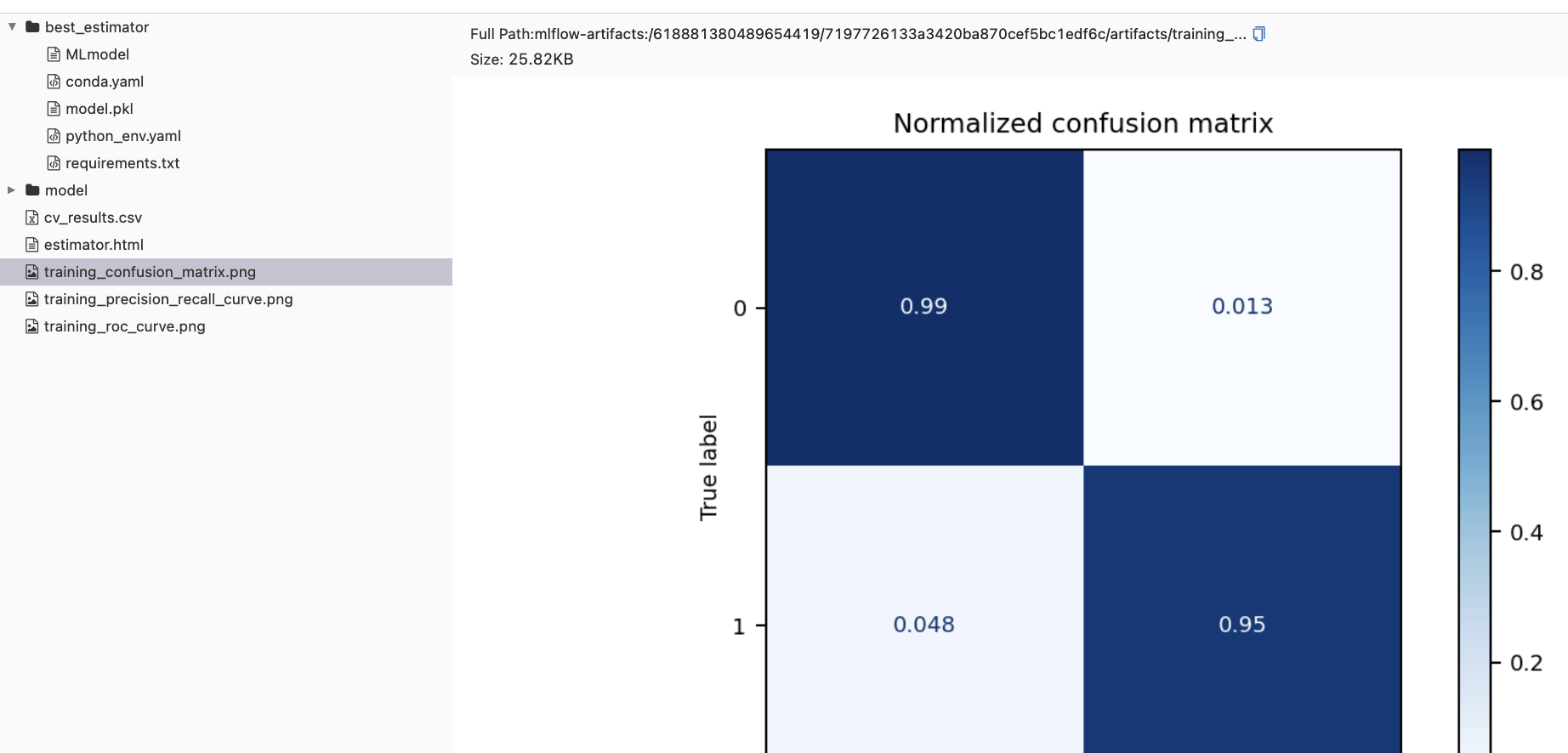`cv_results` was also returned and the logged metrics from all child runs were similar to `cv_results`.

### Test 2. sklearn autolog and dask-ml tuner

``client = Client(processes=False, threads_per_worker=4, n_workers=1)``
``````with mlflow.start_run(run_name="test_2") as run:

search_cv = dask_RandomizedSearchCV(pipe, param_space, cv=ps, n_iter=n_search)
search_cv.fit(
X_train_val,
y_train_val,
clf__eval_set=[(X_val, y_val)],
clf__eval_names=["val"],
clf__eval_metric=["binary_logloss"],
)``````
`````` val's binary_logloss: 0.6207 val's binary_logloss: 0.6207
 val's binary_logloss: 0.64539

 val's binary_logloss: 0.603905
 val's binary_logloss: 0.561648
 val's binary_logloss: 0.561648
 val's binary_logloss: 0.566663
 val's binary_logloss: 0.51229
 val's binary_logloss: 0.51229
 val's binary_logloss: 0.533792
 val's binary_logloss: 0.470843
 val's binary_logloss: 0.470843
 val's binary_logloss: 0.504106
 val's binary_logloss: 0.43163
 val's binary_logloss: 0.43163
 val's binary_logloss: 0.476199
 val's binary_logloss: 0.399279
 val's binary_logloss: 0.399279
 val's binary_logloss: 0.452345
 val's binary_logloss: 0.371128
 val's binary_logloss: 0.371128
 val's binary_logloss: 0.430183
 val's binary_logloss: 0.345312
 val's binary_logloss: 0.345312
 val's binary_logloss: 0.409486
 val's binary_logloss: 0.323905
 val's binary_logloss: 0.323905
    val's binary_logloss: 0.388993
    val's binary_logloss: 0.303382
    val's binary_logloss: 0.303382
 val's binary_logloss: 0.642694
 val's binary_logloss: 0.599408
 val's binary_logloss: 0.5599
 val's binary_logloss: 0.525228
 val's binary_logloss: 0.490788
 val's binary_logloss: 0.461964
 val's binary_logloss: 0.433798
 val's binary_logloss: 0.409936
 val's binary_logloss: 0.385757
    val's binary_logloss: 0.365051``````

`mlflow.sklearn.autolog` still created confusion matrix, ROC curve, and PR curve but only a single run is returned, and all child runs are now missing. Besides, the UI also didn’t log `cv_results`.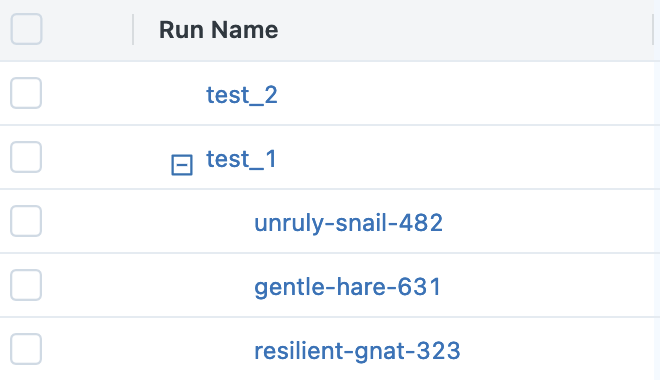#### Is the only logged run the best run?

Here was where the behavior of `mlflow.sklearn.autolog` changed. It was supposed to return a single parent run and multiple child runs but when `dask-ml` was used as tuner, it only logged a single run. I didn’t know whether thi was the best run or not, so I decided to compare the MLflow logged result with the actual search result.

``mlflow_run = mlflow_client.get_run(run.info.run_id)``
``````assert str(search_cv.best_estimator_.get_params()['clf__learning_rate']) == \
mlflow_run.data.params['clf__learning_rate']
assert str(search_cv.best_estimator_.get_params()['clf__subsample']) == \
mlflow_run.data.params['clf__subsample']``````

Luckily, the assertions have passed, meaning that the single recorded run by MLflow was the best run. Except that users can’t see the child runs in the UI, this behavior seems acceptable.

### Test 3. lightgbm+sklearn autolog and sklearn tuner

This test idea came to my mind becasue I imagined it would be very convenient if one could use autologging on top of a sklearn tuner. Thus, I decided to turn on lightgbm autologging in addition to the sklearn autologging.

``mlflow.lightgbm.autolog()``
``````with mlflow.start_run(run_name='test_3') as run:

search_cv = RandomizedSearchCV(pipe, param_space, cv=ps, n_iter=n_search)
search_cv.fit(
X_train_val,
y_train_val,
clf__eval_set=[(X_val, y_val)],
clf__eval_names=["val"],
clf__eval_metric=["binary_logloss"],
)``````
`````` val's binary_logloss: 0.64539
 val's binary_logloss: 0.603905
 val's binary_logloss: 0.566663
 val's binary_logloss: 0.533792
 val's binary_logloss: 0.504106
 val's binary_logloss: 0.476199
 val's binary_logloss: 0.452345
 val's binary_logloss: 0.430183
 val's binary_logloss: 0.409486
    val's binary_logloss: 0.388993
 val's binary_logloss: 0.6207
 val's binary_logloss: 0.561648
 val's binary_logloss: 0.51229
 val's binary_logloss: 0.470843
 val's binary_logloss: 0.43163
 val's binary_logloss: 0.399279
 val's binary_logloss: 0.371128
 val's binary_logloss: 0.345312
 val's binary_logloss: 0.323905
    val's binary_logloss: 0.303382
 val's binary_logloss: 0.632926
 val's binary_logloss: 0.582412
 val's binary_logloss: 0.538314
 val's binary_logloss: 0.500565
 val's binary_logloss: 0.467006
 val's binary_logloss: 0.436234
 val's binary_logloss: 0.410759
 val's binary_logloss: 0.384813
 val's binary_logloss: 0.361938
    val's binary_logloss: 0.342656
 val's binary_logloss: 0.642694
 val's binary_logloss: 0.599408
 val's binary_logloss: 0.5599
 val's binary_logloss: 0.525228
 val's binary_logloss: 0.490788
 val's binary_logloss: 0.461964
 val's binary_logloss: 0.433798
 val's binary_logloss: 0.409936
 val's binary_logloss: 0.385757
    val's binary_logloss: 0.365051``````

This time, I found that sklearn autologging behaved normally but lightgbm autologging didn’t work at all. First, lightgbm autologging metrics such as feature importance scores were missing:Second, `training-log_loss` wasn’t logged for every iteration but it was logged as a single numeric value, and thus was visualized as a bar graph: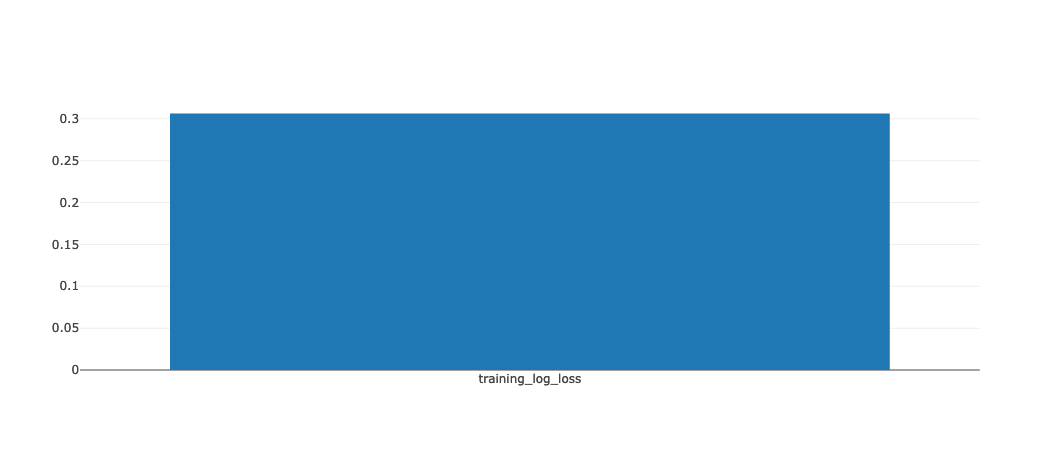### Test 4. lightgbm+sklearn autolog and dask-ml tuner

Finally, I used the dask-ml tuner, lightgbm and sklearn autologging altogether.

``````with mlflow.start_run(run_name='test_4') as run:

search_cv = dask_RandomizedSearchCV(pipe, param_space, cv=ps, n_iter=n_search)
search_cv.fit(
X_train_val,
y_train_val,
clf__eval_set=[(X_val, y_val)],
clf__eval_names=["val"],
clf__eval_metric=["binary_logloss"],
)``````
`````` val's binary_logloss: 0.632926   val's binary_logloss: 0.653833

 val's binary_logloss: 0.632926
 val's binary_logloss: 0.582412
 val's binary_logloss: 0.618878
 val's binary_logloss: 0.582412
 val's binary_logloss: 0.586995
 val's binary_logloss: 0.538314
 val's binary_logloss: 0.538314
 val's binary_logloss: 0.558061
 val's binary_logloss: 0.500565
 val's binary_logloss: 0.500565
 val's binary_logloss: 0.531579
 val's binary_logloss: 0.467006
 val's binary_logloss: 0.467006
 val's binary_logloss: 0.507355
 val's binary_logloss: 0.436234
 val's binary_logloss: 0.436234
 val's binary_logloss: 0.485109
 val's binary_logloss: 0.410759
 val's binary_logloss: 0.410759
 val's binary_logloss: 0.463636
 val's binary_logloss: 0.384813
 val's binary_logloss: 0.384813
 val's binary_logloss: 0.444977
 val's binary_logloss: 0.361938
 val's binary_logloss: 0.361938
    val's binary_logloss: 0.427695
    val's binary_logloss: 0.342656
    val's binary_logloss: 0.342656
 val's binary_logloss: 0.651621
 val's binary_logloss: 0.615177
 val's binary_logloss: 0.581242
 val's binary_logloss: 0.550942
 val's binary_logloss: 0.522853
 val's binary_logloss: 0.494685
 val's binary_logloss: 0.470868
 val's binary_logloss: 0.447008
 val's binary_logloss: 0.42506
    val's binary_logloss: 0.406164``````
``2023/03/19 23:02:26 WARNING mlflow.utils.autologging_utils: Encountered unexpected error during sklearn autologging: The following failures occurred while performing one or more logging operations: [MlflowException('Failed to perform one or more operations on the run with ID 4f0ea51beb0748139aa4364c5d332279. Failed operations: [MlflowException("API request to http://127.0.0.1:5000/api/2.0/mlflow/runs/log-batch failed with exception HTTPConnectionPool(host=\'127.0.0.1\', port=5000): Max retries exceeded with url: /api/2.0/mlflow/runs/log-batch (Caused by ResponseError(\'too many 500 error responses\'))")]')]``

This time, similar to Test 2, a single run was returned but it seemed that lightgbm autologging actually worked because the UI generated images from both sklearn and lightgbm autologging methods: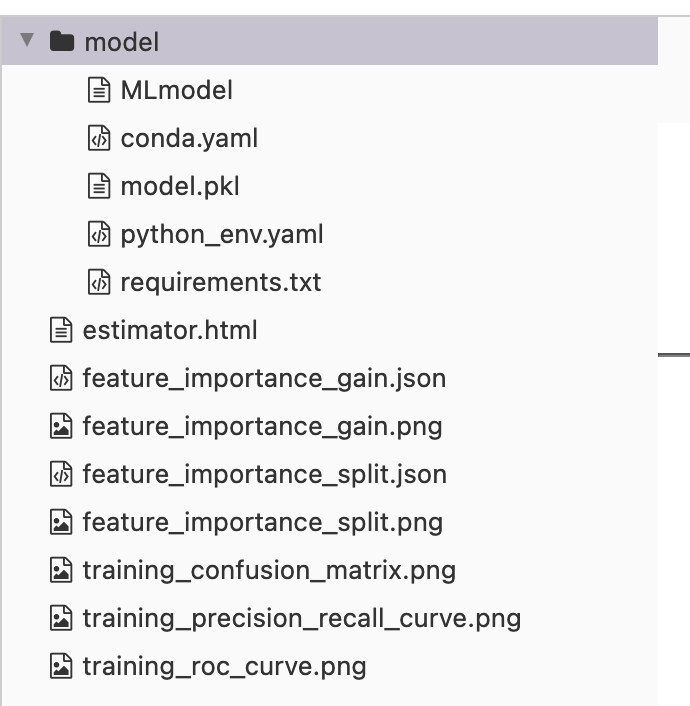However, only single run was returned, again like in Test 2. Unfortunately, this time, this single run didn’t pass the assertion test.

``mlflow_run = mlflow_client.get_run(run.info.run_id)``
``````assert str(search_cv.best_estimator_.get_params()['clf__learning_rate']) == \
mlflow_run.data.params['learning_rate']
assert str(search_cv.best_estimator_.get_params()['clf__subsample']) == \
mlflow_run.data.params['subsample']``````
``AssertionError: ``
``````print(str(search_cv.best_estimator_.get_params()['clf__learning_rate']),
mlflow_run.data.params['learning_rate'])
print(str(search_cv.best_estimator_.get_params()['clf__subsample']),
mlflow_run.data.params['subsample'])``````
``````0.05 0.07777777777777778
0.5 0.7000000000000001``````

This means that when dask-ml, sklearn autolog, and lightgbm autologgin are used at once, we cannot trust the MLflow tracking UI becasue the single set of represented hyperparameters in the UI are not the best estimator’s hyperparameters. This means this combination gives unreliable results, which we should avoid at all costs.

``client.close()``

## Conclusions

In this notebook, I demonstrated how different combinations of autologging and tuners could produce different results. Some of these changed behaviors were simple omissions but I found a more troubling combination as well where the results were just simply wrong. This suggests that when it comes to testing interoperability, we should not only check whether they work together but also whether the returned results are accurate.